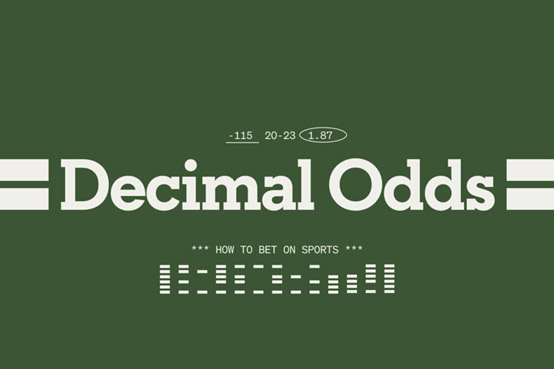# Decimal Odds explainedIf you are a bettor living in America, then you are probably more familiar with the moneyline odds.

If you are a bettor living in UK, then you are probably more familiar with the fractional odds.

If you are a bettor betting from Europe, Australia, New Zealand or Canada, you are probably more familiar with the decimal odds.

In powered by Betfair exchanges, such as Orbit Exchange, all odds are offered in decimals, therefore it is very important that every bettor understand them.

Before we go over in detail what are the decimal odds and why they are widely used n betting exchanges comparing to other odds forms, let’s take a look at a very simple example.

If you were given two fractions, 9/14 and 5/9, could you tell instantly which price is the biggest?

Even if you were very familiar with fractions, it would take you some seconds to figure out which odd is better.

Now, if you were given two numbers, 1.64 and 1.56, you would instantly be able to see that 1.64 is bigger than 1.56.

To understand decimal odds we need to think of them in monetary terms.

1.64 means that if I bet 1€, I will get a 1.64€ return.

1.56 means that if I bet 1€, I will get a 1.56€ return and so on.

Decimal Odds are very commonly used online, mainly because it is easier to understand them comparing to fraction odds and the American moneline odds system. But this is not the only reason. Let’s dig further…

Why are Orbit Exchange odds in decimals?

1) Decimal odds are easy to understand

Consider the example above from the average person’s point of view.

2) Decimal odds provide more options

Decimals allow smaller increments and better competition for prices. For example, instead of 2.5 (6/4), you could ask for 2.52, 2.54, 2.56 or 2.58, which are all available on the Exchange before the next standard fraction of 2.6 (8/5).

3) It is easier to calculate the return with decimal odds
Fractional odds represent the profit

When we see 3/2 fractional odds, we need to read them as

(how much i win) / (how much i bet)

3/2 means you will win 3€ for every 2€ staked = you will win 1.5€

This is your profit. It does not include your stake, which you also get back if you win the bet.
Your total return will be 3/2+1=2.5€

Fractional odds 3/2 and you bet 10€.

Stake x fraction = profit 10×3/2=15. Therefore, your profit is 15€ for a 10€ staked bet.

In order to calculate your total return

[(stakexfraction) + stake] = (10×3/2) + 10 = 15 + 10 = 25€

Decimal odds represent the return

When you see 2.5 decimal odds, you need to read them as

what I will receive in total (including my stake) if I stake 1€

2.5 means your total return if you stake 1€ will be 2.5€

Decimal odds 2.50 and you bet 10€

Odds x stake = total return
2.50 x 10 = 25€.
If you want to calculate your net profit
Total return – stake = net profit
25 – 10 = 15€

As it is clearly deducted from the above, calculating your return is easier with decimal odds, as you need to apply more math if you use the fractional.

Now that you understood what is Orbit Exchange in a nutshell and how the decimal odds work, let’s work out how to navigate through the Orbit Exchange platform and how to read it.